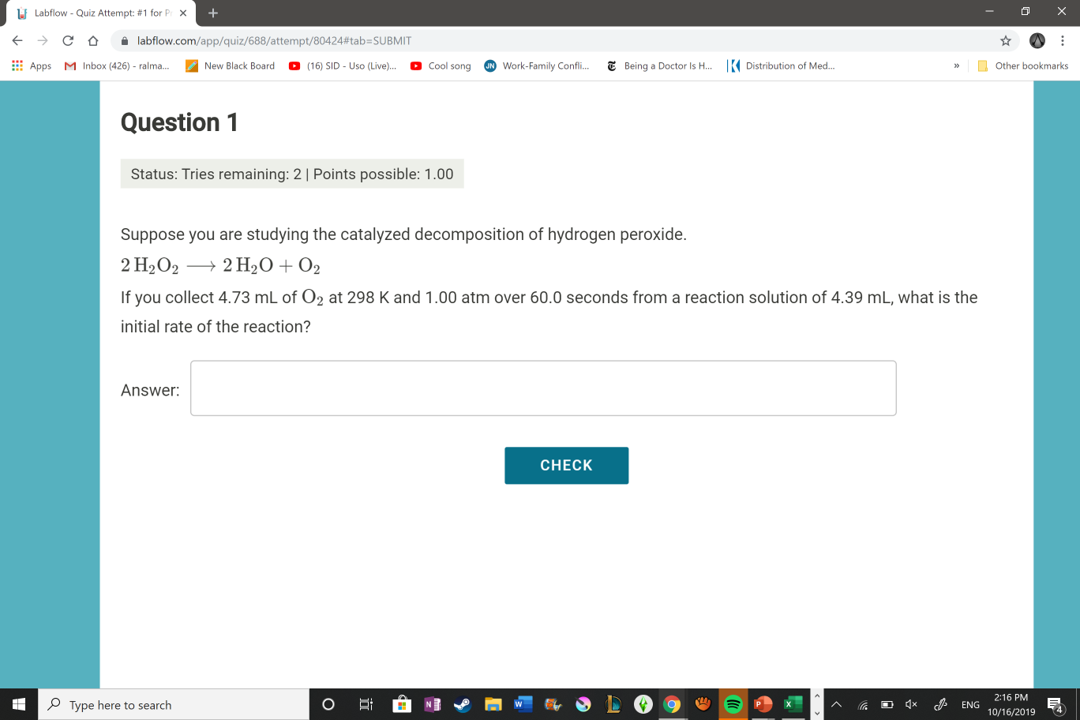# Suppose you are studying the catalyzed decomposition of hydrogen peroxide. 2H2O2⟶2H2O+O2. If you collect a 4.73 mL of O2 at 298 K and 1.00 atm over 60.0 seconds from a reaction solution of 4.39 mL, what is the initial rate of the reaction? So I know that the Rate law indicates that rate= -1/a * delta A / delta t = 1/b * delta B/ delta t. I'm not sure how to implement it into this question. So far I think it could be: -1/2 * delta H2Os / delta t = 1/2 * delta H2O / delta t = delta O2 / delta t. What is delta in this case.